ENGR338 Digital Electronics 2021 Spring
Lab 5 - The Inverter
Name: Ryan Jeanes

Email: rejeanes@fortlewis.edu

The Inverter
Introduction

The inverter is a simple circuit that can be built using nmos and pmos transistors to take an input voltage and output its inverse.

Methods

We created the schematic, icon, and layout of an inverter with pmos having dimensions of 20/2 and nmos dimensions of 10/2. After verifying the design works using LTSpice simulations, we then created another inverter with pmos dimenisons of 100/2 and nmos dimensions of 50/2. We also simulated the 100/50 inverter using LTSpice, then build a circuit using both inverters and simulated the circuit using the ALS and ISRM tools.

Results

All schematics and layouts passed DRC, NCC, and well checks. The 20/10 inverter was tested with a 100fF, 1pF, and 10pF to test its driving capability using a pulse function.  The 20/10 inverter started having some  difficulty driving the 1pF capacitor, and couldn't drive the 10pF capacitor.  With the wider 100/50  inverter replacing the 20/10 inverter the same simulations were run using the same capacitor loads for each simulation. The 100/50 inverter was able to drive the 100f with tiny delay, and had a slightly larger time delay with the 1pF capacitor. The time delay with the 10pF capacitor was more significant but the inverter was still able to drive the load. The last simulations were ran using ALS and ISRM on a circuit containing both inverters in parallel driving 100fF loads.Figure 1 - Schematic of the 20/10 inverter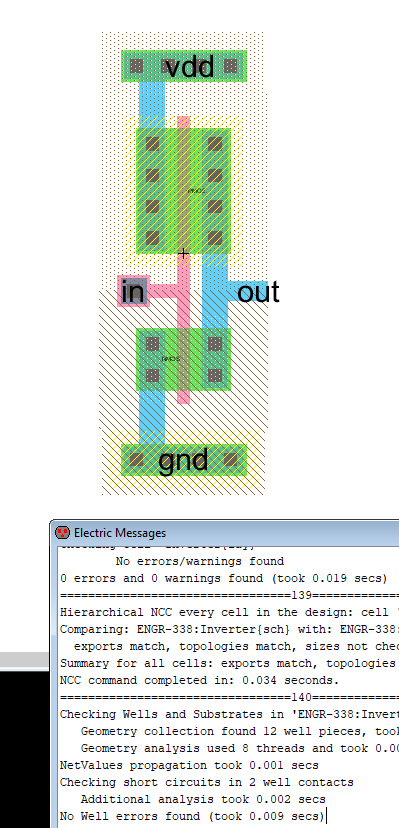Figure 2 - Layout of inverter with passed checks.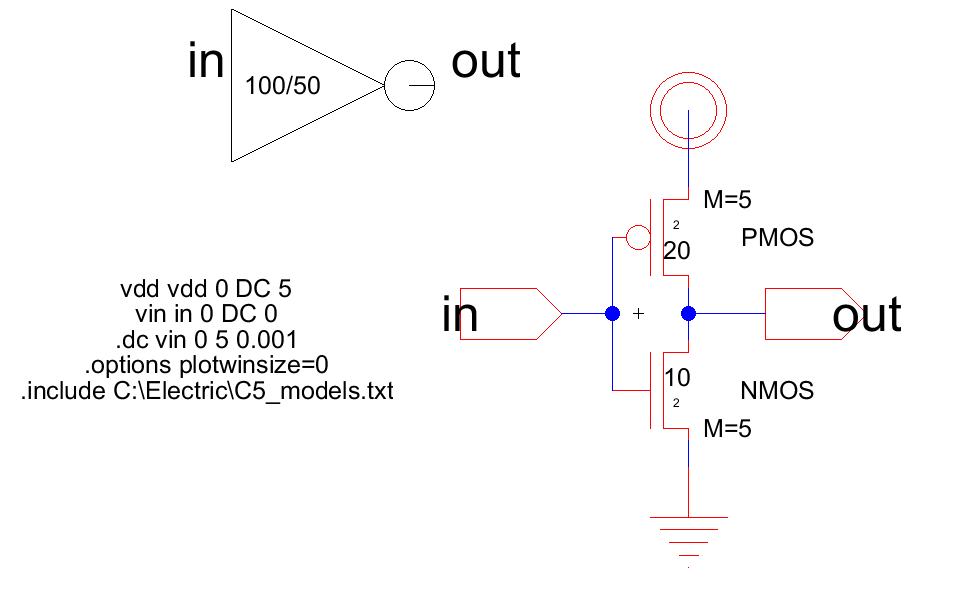Figure 3 - 100/50 schematic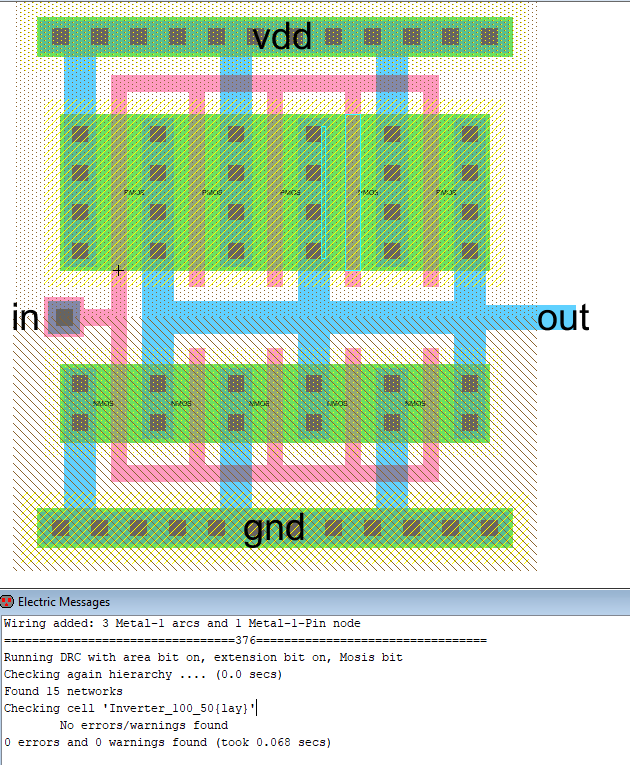Figure 4 - Layout of the 100/50 inverter with passed DRC checks.Figure 5 - Test simulation of the inverter.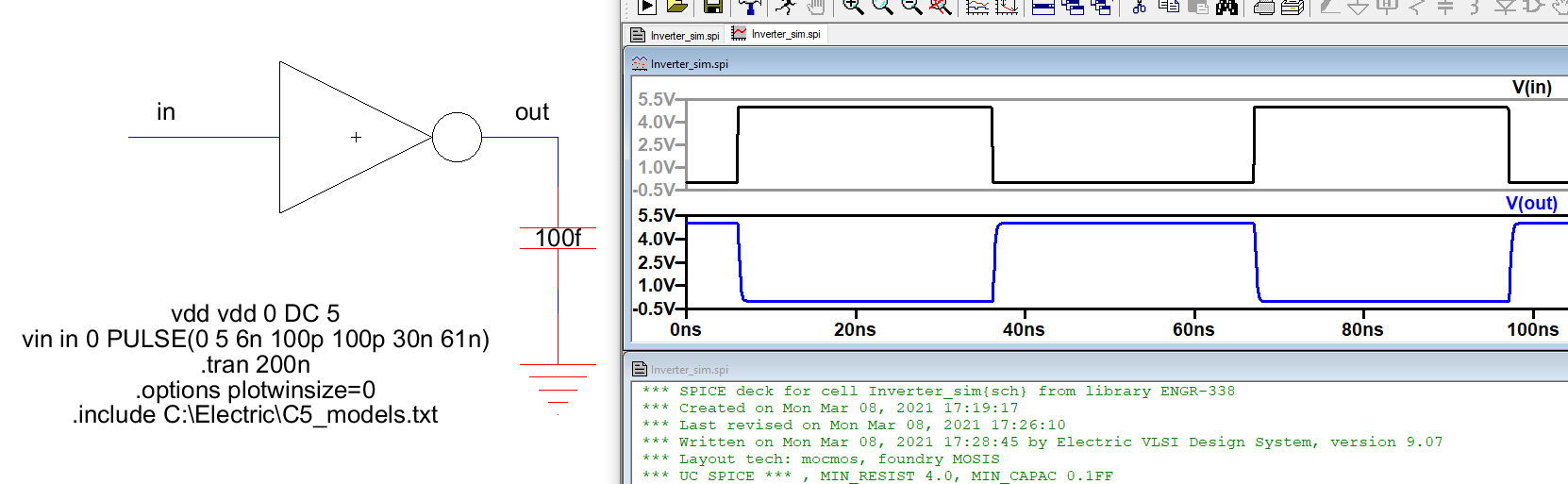Figure 6 - Simulating the 20/10 inverter driving a 100fF load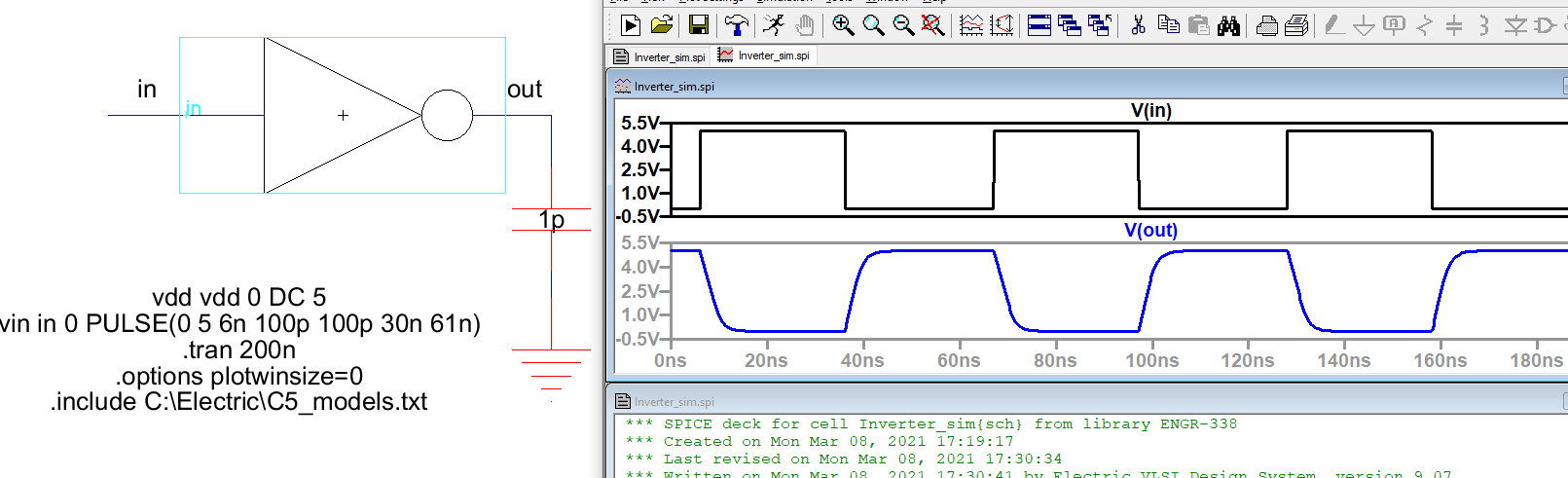Figure 7 - Simulating the 20/10 inverter driving a 1pF load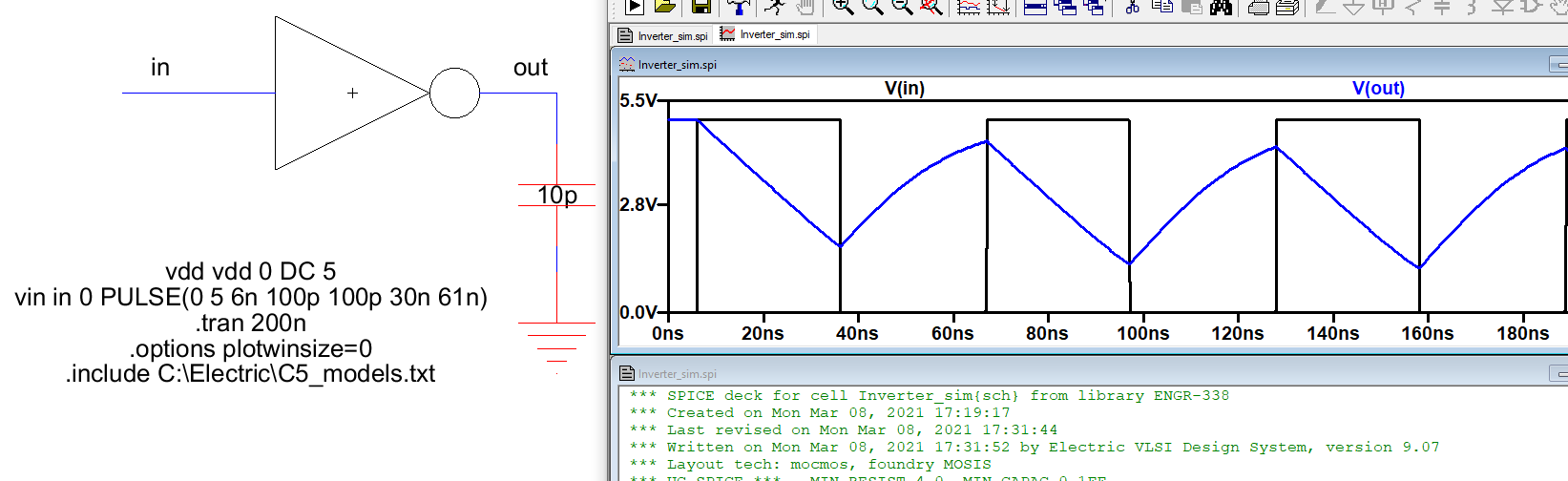Figure 8 - Simulating the 20/10 inverter driving a 10pF load. The inverter is unable to drive the 10pF load.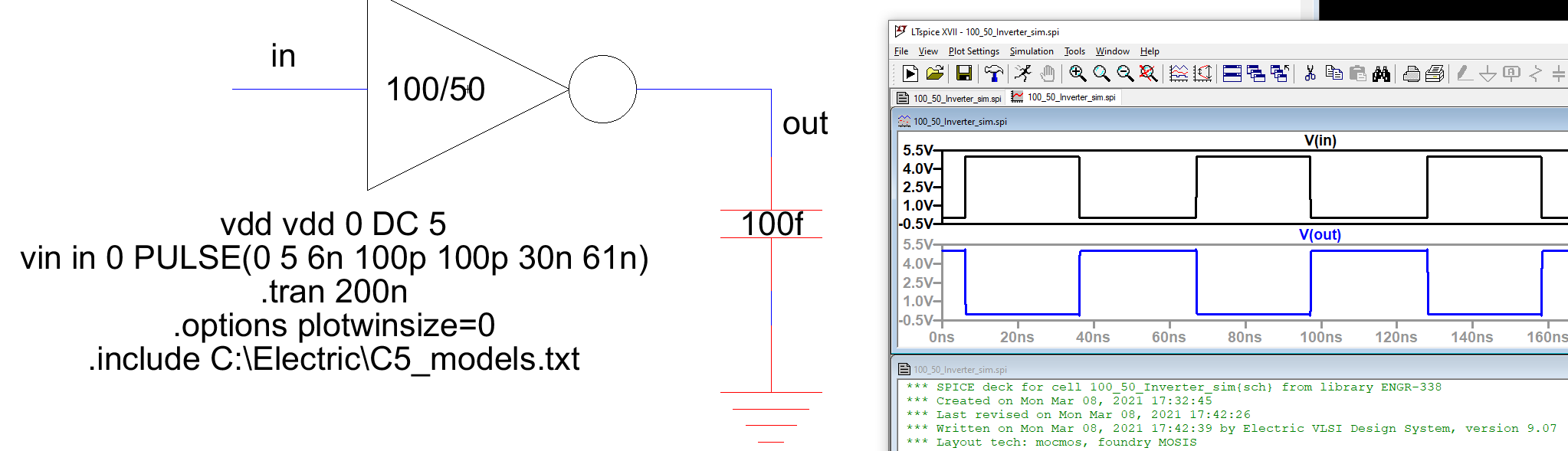Figure 9 - Simulating the 100/50 inverter driving the 100fF load.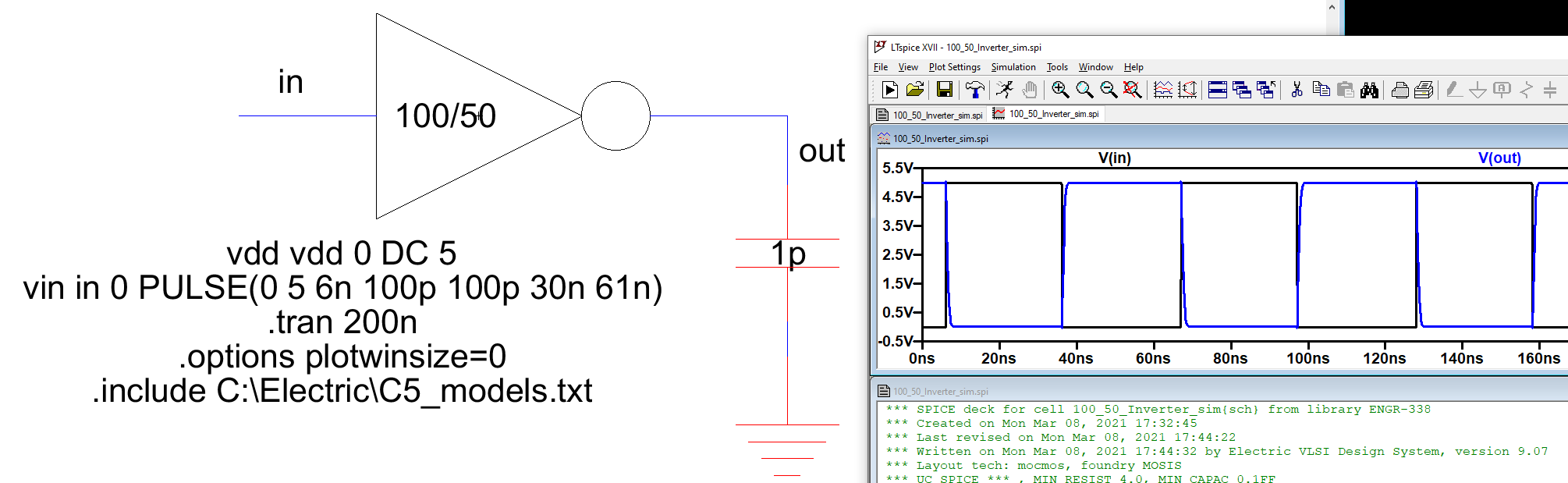Figure 10 - Simulating the 100/50 inverter driving the 1pF load, showing some slight time delay.Figure 11 - Simulating the 100/50 inverter driving the 10pF load, with more prevalent delay shown.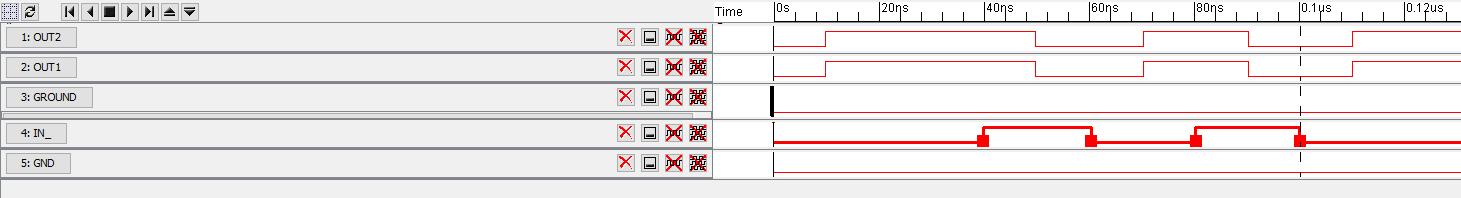Figure 12 - ALS simulation showing the time delays of both the outputs.Figure 13 - ISRM simulation showing the time delay with both voltage outputs.

Discussion

The schematics and layouts were built without any problems. The interesting thing with the simulations using the ALS and ISRM tools is that the ALS simulation showed an approximate 10ns time delay when the input voltage switched to high, while the ISRM tool showed a 0.1ns time delay when the input voltage switched to high.